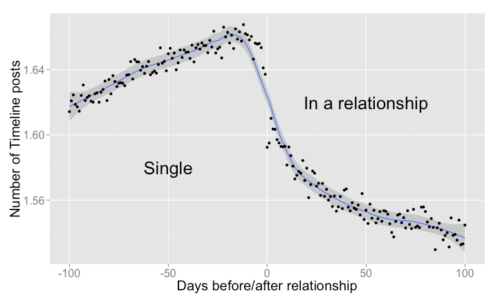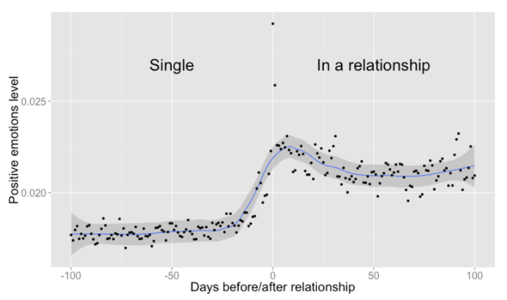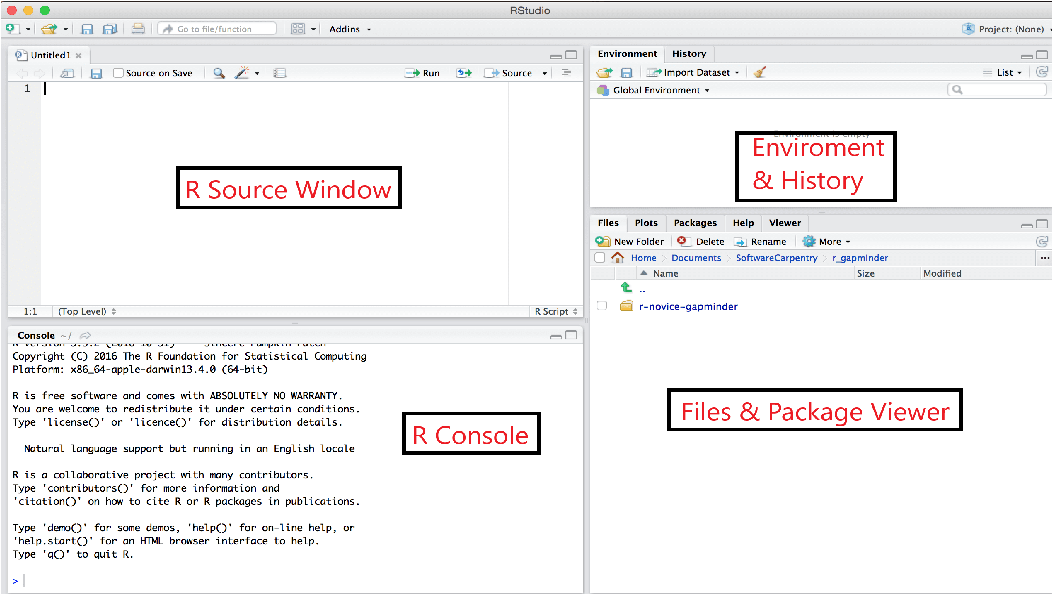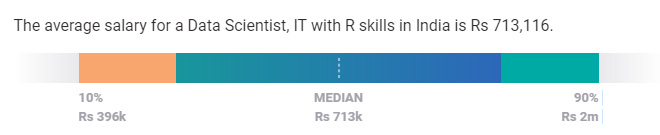# What is R Programming?In this article on What is R programming, I’ll be concentrating on explaining the basic concepts of R.

There are 2.72 million jobs available in the field of data science. R and Python are the two pillars that make playing with data easier. In this article on What is R programming, I’ll be concentrating on explaining the basic concepts of R.

I will cover the following topics in this blog:

• Features of R
• Installing R & RStudio
• R package & libraries
• Variables & Data types
• Operators
• Conditional statements
• Looping statements
• Control Statements
• Functions
• Scope of R Programming

R is an open-source tool used for statistics and analytics. It has become popular in recent years with its applications in the field of Data Analytics, Data Science and Machine Learning among others.

Before we get into features and basics of R Programming, let’s see a scenario where R is used in companies.

Facebook, an online social media-based company aims at improving user engagement, creating and sharing posts. It uses R for exploratory analysis, user engagement analysis, etc. Facebook Data Science group had released a series of blogs that showed an analysis of timeline posts made by users who were Single versus those In a Relationship. The following graph shows the average number of timeline posts exchanged between two people who are about to become a couple.The above graph shows the steady change in the number of timeline posts 100 days before and after the relationship. The below graph shows the positive emotions increasing by using tags, words expressing positive emotions.Now that we have an idea of what is R, let’s move onto the features of R.

## Features of R

Features of R are:

• It is an open-source tool
• R supports Object-oriented as well as Procedural programming.
• It provides an environment for statistical computation and software development.
• Provides extensive packages & libraries
• R has a wonderful community for people to share and learn from experts
• Numerous data sources to connect.

Let’s move ahead to install R and RStudio.

## Installing R & RStudio

Go to the R download page and click on the respective OS, *click on *base subfolder. You will find the downloadable link on the top of the page. Run the .exe file and complete the installation by pressing next and install. When you run the R Gui app, the R Console page will be visible at the start.

RStudio is an IDE used for R Programming which is available as open-source and commercial software for Desktop and Server products. Download RStudio Desktop from the RStudio downloads page. On the successful download of the file, run the .exe file and complete the installation. Open the RStudio App and you will see that the entire window is divided into 4 panes as below.### Source window

• We add the source code here and run the whole code by clicking on the source button. To run selected lines, select lines and click Ctrl + Enter or Run button. Run a single line by clicking on CTRL+ Enter.

### R Console

• R displays error logs, warnings, executed statements with their outputs in this pane.

### Environment and History

• This pane consists of 3 tabs. The Environment tab displays all variables defined and used in the R session. The history tab displays the executed statements in R source and Console. The Connections tab display database and external connection-related information.

### Files & Package Viewer

• This pane consists of 5 tabs. The Files tab displays the files in the current working directory. The Plots tab displays graphs, charts created using R packages. The Packages tab lists down installed packages. It also contains 2 buttons (install and update). The Help tab displays the documentation of any package or function in R. The Viewer tab displays web applications and maps that are created using R.

Note: In case any of the 4 panes are closed or hidden, Go to View -> Panes -> Show All Panes to view all panes.

Let’s move forward to learn what is a package and how to load the packages in RStudio.

## R package & Libraries

R packages are a group of functions bundled together. These functions are pre-compiled and used in R scripts by preloading them. As discussed above, we can find the list of packages installed in the packages tab at the bottom right window. Let’s learn how to install packages in RStudio.

To install a package, use the following syntax in R Source or R Console.

`install.packages([package-name])`

By default, RStudio installs the packages from CRAN Repository. We can use the functions by loading the package into memory.

To load the package, use the following syntax.

`library([package-name])`

Try Installing the dplyr package in your system and find out what is it used for.

## Variables & Data types

### R Variables

Variable is the name of the memory location where data is stored. In other words, we can access memory data using variables.

In R, we can assign variables using any of the following syntaxes. The below-mentioned example assigns the value Edureka to the variable Company.

• Company = “Edureka”
• company <- “Edureka”
• “Edureka” -> CompanY

Note: R variables are case-sensitive.

Variables can be categorized into Continuous and Categorical. If a variable can take on any value between its minimum value and its maximum value, it is called a Continuous variable. Categorical variables (sometimes called a nominal variable) are those that have a fixed number of values or choices such as “Yes”, “No”, etc.

### Datatypes

R consists of 5 main data types: List, Data frame, Vector, Array and Matrix. There are 2 other types called factor and tibble, which are not primary datatypes but will be discussed below.Let’s discuss all the data types in detail.

• List
• Vector
• Array
• Matrix
• Dataframe
• Tibble
• Factor

#### List

A list holds a list of elements. These elements could include either number, decimal number, character, or Boolean value (True/False). They are mutable, i.e., the elements in a list can be modified using the index. A list can also contain a combination of lists, vector, array, and matrix. Let’s learn various list operations –

• Creating a list List is created using list( ) function. Use the following syntax to create a list. `list(val1,val2, . . . )`
• Example:*
``````mylist_1 = list(1, 3.14, "abc", "x")
mylist_1``````
• Output:*
``````[]
 1
``````

[]  3.14

[]  "abc"

[]  "x

``*   You can create a nested list using the same list( ) function. The only difference is that a nested list can have numbers, characters, lists, and other datatype variables.``

nested_list = list(1,mylist_1,list(1,5,"a"))

``````
_Try adding symbols ( \$ . / & ) into a list. [Hint: Escape characters]_

Note : Check the data type of variable using class(variable_name).

#### Display list

*   Display or print list elements by calling the **print( )** function or simply list name.
**Example:**``````

names = list("Rahul","Nikita","Sindhu","Ram") names

``**Output:**``

[]  "Rahul"

[]  "Nikita"

[]  "Sindhu"

[]  "Ram"

``````*   **Accessing List Elements**
We access each element within a list using an index. Let’s see some examples of how to access elements.
**Example:**``````

#Create a list of names. names = list("Rahul","Nikita","Sindhu","Ram") #Access first element. names

``**Output:**``

[]  "Rahul"

``````*   Subsetting is the process of accessing several elements. The **subset** function is used to return **subsets** of a vector, matrix, or data frame which meets a particular condition. _R_ has powerful indexing features for accessing object elements. These features can be used to select and exclude variables and observations.
_The index of an R variable starts from 1 to the length of the list._
**Example:**``````

#uisng : names[2:3] #using vector method. names[c(2,3)]

``**Output:**``

[]  "Nikita"

[]  "Sindhu"

``````
#### Update list

*   Existing elements in a list can be updated by using the element index. Update list elements by assigning a new value to an existing element.
**Example:**``````

#Update 3rd name in names from Sindhu to Shreya. names = "Shreya" names

``**Output:**``

[]  "Rahul"

[]  "Nikita"

[]  "Shreya"

[]  "Ram"

``````

*   As discussed before, lists are mutable, i.e. list elements can be added as well as be updated. Add a new element into a list using list function or using the length function.
**Example:**``````

names = "Seetha" names

``**Output:**``

[]  "Rahul"

[]  "Nikita"

[]  "Sindhu"

[]  "Ram"

[] NULL

[]  "Seetha"

``````*   Did you see something different from the previous output? That brings us to a question **What is NULL**?
*   NULL represent an element with zero length. Use length function to find the last index and add the element to the list.``````

names[length(names)+1] = "Edureka" names

``*   **Output:**``

[]  "Rahul"

[]  "Nikita"

[]  "Bindhu"

[]  "Ram"

[]  "Edureka"

``````
_Try to add NULL into a list at any desired position_

*   **Delete elements**
*   List elements can be deleted by assigning the element to **NULL**.
**Example:**``````

#Delete list elements names = NULL names

``*   **Output:**``

[]  "Rahul"

[]  "Nikita"

[]  "Sindhu"

``````
_Most of you would have noticed [[ ]] and [ ] in list outputs. Find what is the difference between [[ ]] and [ ]._

#### Vector

![What is R Programming](https://d1jnx9ba8s6j9r.cloudfront.net/blog/wp-content/uploads/2019/09/04-298x300.png "What is R Programming")

**A vector** is like a list but stores similar types of data, i.e. Numeric, characters or strings, etc. It converts all the elements into a single type depending on the elements in the vector. We can categorize a vector into the below types as shown in the image.

*   **Numeric Vector** (1,808,6527,742,268)
*   **Integer Vector** ( positive and negative real numbers )
*   **Character vector** (“a”, “efjvfVF”, “fbyvkdsb sbv”, “ffWVWVVRV”)
*   **Logical vector** (True/False)
*   **Complex vector** (complex numbers of a+bi form)

Let’s learn vector operations.

### Vector Operations

*   **Create a vector**
*   Create a vector using c( ) function. Use the following syntax to create a vector.
`c(val1, val2, ....)```````

Roll_no = c(1,2,3,4,5) Roll_no

``**Output:**``

 1 2 3 4 5

``````
Note: R has built-in constants. Ex: letters[1:3] = {“a” “b” “c”}, LETTERS[1:3] = {“A” “B” “C”}

The rest operations are the same as a list which brings us to the question: **What is the difference between a list and a vector?**

#### Difference between list and a vector

*   A list holds different data such as Numeric, Character, logical, etc. Vector stores elements of the same type or converts implicitly.
*   Lists are **recursive, **whereas vector is not.
*   The vector is one-dimensional, whereas the list is a multidimensional object.

#### Array

**Array** store data in more than two dimensions. It takes vectors as input and uses the values in the dim parameter to create an array.

The basic syntax for creating an array in R is −

`array(data, dim, dimnames)`

Where,

*   `data` input vector which becomes the data elements of the array
*   `dim` the dimension of the array, where you pass the number of rows, column and the number of matrices to be created by mentioned dimensions
*   `dimname` are the names assigned to the rows and columns

**Example:**``````

v1 = c(9,1,3) v2 = c(1,7,9,6,4,5) #Take these vectors as input to the array. result = array(c(v1,v2),dim = c(3,3,2)) result

``````
**Output:**``````

, , 1 [,1] [,2] [,3] [1,] 9 1 6 [2,] 1 7 4 [3,] 3 9 5 , , 2 [,1] [,2] [,3] [1,] 9 1 6 [2,] 1 7 4 [3,] 3 9 5

``````
_What is the difference between NA and NULL?_

Note: Check out the number of rows and columns of R object using nrow(var) and ncol(var).

#### Matrix

A **matrix** is a collection of data elements arranged in a two-dimensional rectangular layout.

The syntax to create a matrix is –

`matrix(data, nrow, ncol, byrow, dimnames)`

Where:

*   `data` is the input vector,
*   `nrow` the number of rows to be created
*   `ncol` is the number of columns to be created
*   `byrow` is a logical clue. If TRUE, then the input vector elements are arranged by row
*   `dimname` names assigned to the rows and columns

**Example:**``````

A = matrix(c(2, 6, 3, 1, 5, 7),nrow=2,ncol=3,byrow = TRUE) A

``````
**Output:**``````
`` [,1] [,2] [,3]``

[1,] 2 6 3 [2,] 1 5 7

``````
#### Data Frame

A **Data Frame** is a table-like structure that contains rows and columns. A data frame can be created by combining vectors.

The basic syntax for creating a data frame using is

`data.frame(vect1, vect2, ...)`

**Example:**``````

id = c(1:5) names = c("Srinath","Sahil","Anitha","Peter","Siraj") employees = data.frame(Id = id, Name = names) employees

``````
**Output:**``````

Id Name 1 1 Srinath 2 2 Sahil 3 3 Anitha 4 4 Peter 5 5 Siraj

``````
#### Characteristics of a data frame

*   The column names should be non-empty
*   Each column should contain the same amount of data items
*   The data stored in a data frame can be of numeric, factor or character type
*   The row names should be unique

Note: Check out description of any variable using str(variable)

### Tibble

A **Tibble** is a table-like structure similar to a data frame. Create a tibble variable using the following syntax:

`tibble(list1,list2, ... )`

**Example:**``````

id = c(1:5) names = c("Srinath","Sahil","Anitha","Peter","Siraj") employees = tibble(Id = id, Name = names) employees

``````
**Output:**``````

# A tibble: 5 x 2

`` Id Name``

1 1 Srinath 2 2 Sahil 3 3 Anitha 4 4 Peter 5 5 Siraj

``````
Let’s find out what makes a tibble different from the data frame.

#### Differences between Tibble and Data Frame

*   Tibble displays data along with the data type whereas a data frame display data only
*   Tibble fetches data from the data source in its original data type. Dataframe fetches data from the data source as factors if data types are not specified
*   Tibble is stricter than data frames in slicing. Slicing is a list/vector operation to return a **slice** in a given R object(vector, data frame)

Note: Check out dimensions of any variable using dim(var).

### *actor

A **factor** is another data type that is created while reading data from external data sources. While loading CSV or text files, it converts any column with categorical values to factor. Any vector can be converted to factor using below syntax:

**Syntax:**
``````

as.factor(vector)

``````
A factor converts categorical values into a numerical vector with multiple levels.

**Example:**
``````

as.factor(names)

``````
**Output:**
``````

 Rahul Nikita Sindhu Ram Levels: Nikita Rahul Ram Sindhu

``````
Now we have learned different data types of R. Let’s move ahead and learn about operators in R programming.

## **Operators**

R supports the following operators,

#### **Arithmetic Operators**

![What is R Programming](https://i.imgur.com/422dpRa.png "What is R Programming")

*   **Relational Operators**
![What is R Programming](https://i.imgur.com/iqYnxHR.png "What is R Programming")

*   **Logical Operators**
![What is R Programming](https://i.imgur.com/Sz4L96E.png "What is R Programming")

### Assignment Operators

Assignment operator assigns value or variable to operand.

The assignment operators are =, <-, ->.

**Examples:**``````

10 -> b a = 5 c <- a+b

``````
We have covered different operators used in R Programming, now let’s understand various Conditional, Looping and Control statements.

## **Conditional statements**

R comprises 3 conditional statements which are

![What is R Programming](https://d1jnx9ba8s6j9r.cloudfront.net/blog/wp-content/uploads/2019/09/condition-1.png "What is R Programming")

Lets us discuss them individually.

### **If Statement**

The flow of **If** statement:

![What is R Programming](https://d1jnx9ba8s6j9r.cloudfront.net/blog/wp-content/uploads/2019/09/If-Statement-287x300.jpg "What is R Programming")

As shown in the above picture, if the condition is true, then execute **If code** else executes the statements that come after if body.

**Syntax:**

`if(condition) {`

`If code`

`}`

`statements`

**Example:**``````

``````
**Output:**``````

 "Good"

``````
### Else If Statement

The flow of **Else If** Statement:

![What is R Programming](https://d1jnx9ba8s6j9r.cloudfront.net/blog/wp-content/uploads/2019/09/else-flow-413x300-1.jpg "What is R Programming")

As shown in the above picture, if the condition is true, then execute **If code** else executes **Else code** and then follow the statements that come after the if-else body.

**Syntax:**

`if(condition) {`

`If code`

`}`

`else {`

`Else code`

`}`

`Statements`

**Example:**``````

``````
**Output:**``````

 "Good"

``````
### **If Else If Statement**

The flow of If Else If Statement:

![What is R Programming](https://d1jnx9ba8s6j9r.cloudfront.net/blog/wp-content/uploads/2019/09/else-flow-413x300.jpg "What is R Programming")

As shown in the above picture, if the condition is true, then execute **If code** else checks the second condition. If the condition is true, execute **Else If code** otherwise executes **Else code **followed by statements that come after if-else-if body.

**Syntax:**

`f(condition) {`

`If code`

`}`

`else if (condition){`

`Else if code`

`}else {`

`Else code}`

**Example:**``````

``**Output:**``

 "Ok"

``````
**Switch statement**

A switch is another conditional statement used in R. If statements are generally preferred over switch statements. The basic syntax of the switch statement is –

**Syntax:**

`switch (expression, list)`

**Example:**``````

switch(2,"GM","GA","GN")

``````
**Output:**``````

 "GA"

``````
## **Looping statements**

Looping statements reduce the work of a user to perform a task multiple times. These statements execute a segment of code repeatedly until the condition is met.

R comprises 3 looping statements which are,

![What is R Programming](https://d1jnx9ba8s6j9r.cloudfront.net/blog/wp-content/uploads/2019/09/02-528x175.png "What is R Programming")

Lets us discuss each in detail.

**For Loop**

For loop is the most common looping statement used for repeating a task. A for loop executes statements for a known number of times. Define a for loop using the following syntax:

**Syntax:**

`for(var in range){`

`statements`

`}`

**Example:**``````

for(x in 1:10){ print(x) }

``````
**Output:**``````

 1  2  3  4  5  6  7  8  9  10

``````
**While Loop**

A **while loop** repeats a statement or group of statements until the condition is true. It tests the condition before executing the loop body. A while loop is created using the following syntax:

**Syntax:**

`while(condition) {`

`Statement`

`}`

**Example:**``````

a = 5 while(a>0) { a=a-1 print(a) }

``````
**Output:**``````

 4  3  2  1  0

``````
#### **Repeat**

Repeat loop is the best example of an exit controlled loop where the code is first executed and then the condition is checked to determine if the control should be inside the loop or exit from it. Create a repeat loop using the following syntax:

**Syntax:**

`repeat {`

`statements`

`if(condition) {`

`statements`

`}`

`}`

**Example:**``````

m=5 repeat { m= m+2 print(m) if(m>15) { break } }

``````
**Output:**``````

 7  9  11  13  15  17

``````
**Control statements**

R has the following control statements,

![What is R Programming](https://d1jnx9ba8s6j9r.cloudfront.net/blog/wp-content/uploads/2019/09/control.png "What is R Programming")

Lets us discuss each in detail.

#### Break

A **break** statement is used to stop or terminate the execution of statements. When the break statement is encountered inside a loop, the loop is immediately terminated and program control resumes at the next statement following the loop. If else and switch statements contain break statements usually to stop the execution. The syntax to use the break statement is –

**Syntax:**

`break`

**Example:**``````

m=5 repeat { m= m+2 print(m) if(m>15) { break } }

``````
**Output:**``````

 7  9  11  13  15  17

``````
#### Next

The **next** statement is used to skip the current iteration of a loop without terminating or ending it. The syntax of the next statement is

**Syntax:**

`next`

**Example:**``````

for(i in c(1:6)) { if (i == "3") { next } print(i) }

``````
**Output:**``````

 1  2  4  5  6

``````
## Functions

A **function** is a set of statements to perform a specific task. R has in-built functions and also allows the user to create their own functions. A function performs a task and returns a result into a variable or print the output in the console.

R contains two types of functions,

![What is R Programming](https://d1jnx9ba8s6j9r.cloudfront.net/blog/wp-content/uploads/2019/09/functions.png "What is R Programming")

#### Built-in Functions

Built-in functions are those pre-defined in R such as mean, sum, median, etc.

#### User-Defined Functions

User-Defined functions are defined as per the requirements. Define a function using the following syntax:

### Function definition

`function_name <- function(arg_1, arg_2, ...) {`

`Function body`

`}`

Store the function definition in a variable and call the function using variable followed by optional parameters inside the parenthesis ( ).

**Example**``````

factorial <- function(n) { if(n<= 1) { return(1) } else { return(n * factorial(n-1)) } } factorial(3)

``````
**Output:**``````

 6

```

## Scope of R programming

In this busy world, everybody learns a new language or technology for the sake of career, fame or salary. Before learning or taking up any course, this question would come to anyone’s mind “What is R Programming and why to learn R over other technologies and tools?”.

R has an excellent growth in various aspects such as Career growth, Job aspect, Business requirements, Cost, Salary, etc. It is open source and has been gaining a lot of audiences lately. It reduces half the burden to buy a licensed product. R is an All in one tool that not only performs analysis but is also used in making reports, dashboards, applications, etc. let’s discuss a few aspects of “why to learn R?’.

### Salary

The need for people with R skills is increasing and so is the salary. Salary of engineers or programmers working with R varies between 3.9LPA to 20LPA. As shown in the image below.Source: Payscale.

### Job roles

The number of jobs available for R Programmers is increasing in recent years. There are different roles available for people with R Programming skills such as:

1. Data Scientist
2. Data Analyst
3. R Programmer/ Developer
5. Data Science Engineer
6. ML Engineer

### Career growth & Job opportunities

According to the various forums, data analysts will be in high demand in companies around the world. R is the most used analytics tool across the world which is estimated to have a wide range of users. Various companies such as Infosys, Wipro, Accenture, etc have grown in this domain to hire talented people as well as provide training to their employees.

## Why You Should Learn R — Learn Data Science with Dataquest

Why should you learn R programming when you're aiming to learn data science? Here are six reasons why R is the right language for you.

## Most popular Data Science and Machine Learning courses — July 2020

Most popular Data Science and Machine Learning courses — August 2020. This list was last updated in August 2020 — and will be updated regularly so as to keep it relevant

## PyTorch for Deep Learning | Data Science | Machine Learning | Python

PyTorch for Deep Learning | Data Science | Machine Learning | Python. PyTorch is a library in Python which provides tools to build deep learning models. What python does for programming PyTorch does for deep learning. Python is a very flexible language for programming and just like python, the PyTorch library provides flexible tools for deep learning.

## Data Augmentation in Deep Learning | Data Science | Machine Learning

Data Augmentation is a technique in Deep Learning which helps in adding value to our base dataset by adding the gathered information from various sources to improve the quality of data of an organisation.

## Difference between Machine Learning, Data Science, AI, Deep Learning, and Statistics

In this article, I clarify the various roles of the data scientist, and how data science compares and overlaps with related fields such as machine learning, deep learning, AI, statistics, IoT, operations research, and applied mathematics.